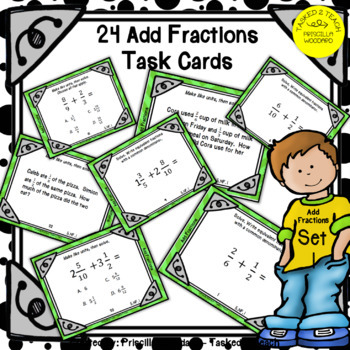Subject
Resource Type
File Type

Zip

Product Rating
Standards
3 Products in this Bundle
3 products
1. Add Fractions with Unlike Denominators: These 24 task cards are aligned to 5.NF.1 to assist your math scholars in mastering adding fractions with unlike denominators. These cards are an introduction to creating equivalent denominators. Students will practice creating fractions with common denomina
2. Add Fractions with Unlike Denominators that are not multiples of each other & multiples of each other: These 24 task cards are aligned to Common Core 5.NF.1. They will assist your math scholars in mastering adding fractions with unlike denominators. Minimal prep...cards are offered in color an
3. Subtract Fractions with Unlike Denominators: These task cards will provide your math scholars with two fractions with unlike denominators. The will write equivalent fractions with a common denominator. Your math scholars will also use equivalent fractions to subtract fractions and mixed numbers with
• Bundle Description
• StandardsNEW

A BUNDLE of Engagement at 30% off!! Engage your scholars with 2 sets of adding fractions task cards and a set of subtracting fractions task cards at a 30% discount off of the original price!!

Set 2 Add Fractions Task Cards: Add Fractions with Unlike Denominators that are not multiples of each other & multiples of each other.

Set 3: Subtract Fraction Task Cards: Allows your math scholars to explore converting unlike denominators to a common denominators to subtract fractions and mixed numbers.

Answer Keys and Recording Sheet for all 3 sets.

Use this task card BUNDLE to DIFFERENTIATE your instruction, small groups, math centers and your early finishers.

Click the PREVIEW to go in for a closer look!!

FEEDBACK

I truly value your feedback. Earn each time you provide feedback!! TPT gives you TPT credit you can use toward lowering the cost of other products.

I would love to send you notifications of sales, new product launches, and freebies.

Click on the GREEN star next to my logo, to follow me. New products are always 50% off for the 1st 24 hours they are posted. It pays to follow me!!

Relish in Teaching,

Priscilla A. Woodard

Solve word problems involving addition and subtraction of fractions referring to the same whole, including cases of unlike denominators, e.g., by using visual fraction models or equations to represent the problem. Use benchmark fractions and number sense of fractions to estimate mentally and assess the reasonableness of answers. For example, recognize an incorrect result 2/5 + 1/2 = 3/7, by observing that 3/7 < 1/2.
Add and subtract fractions with unlike denominators (including mixed numbers) by replacing given fractions with equivalent fractions in such a way as to produce an equivalent sum or difference of fractions with like denominators. For example, 2/3 + 5/4 = 8/12 + 15/12 = 23/12. (In general, 𝘢/𝘣 + 𝘤/𝘥 = (𝘢𝘥 + 𝘣𝘤)/𝘣𝘥.)
Total Pages
Included
Teaching Duration
N/A
Report this Resource to TpT
Reported resources will be reviewed by our team. Report this resource to let us know if this resource violates TpT’s content guidelines.